# Physics 421 M Course CRN 15532 Class 09

• Slides: 58Physics 421 M Course CRN: 15532 Class: 09: 30 -10: 30, MWF, 00 REMOTE Text: Mathematical Methods in the Physical Sciences, 3 rd Ed. , Mary L. Boas https: //pages. uoregon. edu/imamura/421/physics. 421. html Instructor: James Imamura Office: 457 Willamette Hall E-mail: [email protected] edu Phone: 541 -346 -5212 Office Hours: by appointment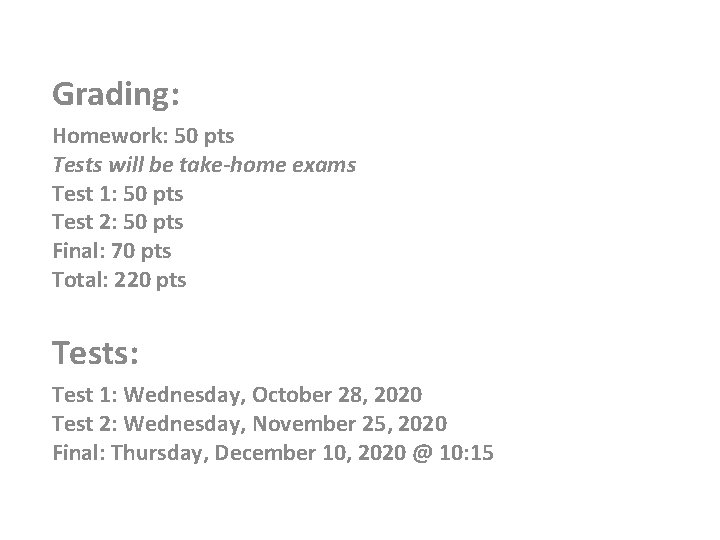Grading: Homework: 50 pts Tests will be take-home exams Test 1: 50 pts Test 2: 50 pts Final: 70 pts Total: 220 pts Tests: Test 1: Wednesday, October 28, 2020 Test 2: Wednesday, November 25, 2020 Final: Thursday, December 10, 2020 @ 10: 15Material: • • • Vector Algebra. Chapters 3. 4, 3. 5, 6. 1 -6. 3: vector analysis and vector operations, addition, subtraction, multiplication (scalar, dot product, cross product), multiplication involving 3 or more vectors (Triple Scalar Product, Triple Vector Product, Laplace's Identity, and applications); vector functions. rotations (Chapter 3. 7) and vectors as first rank tensors (and scalars as zero rank tenors) (Chapter 10. 2). For additional enrichment and enjoyment on other transformations needed for Cartesian vectors, see 10. 6 not to be tested. Vector Calculus. Chapter 6. 4 -6. 12, parts of Chapter 4 (Partial Differentiation), Chapter 5 (Multipole Integrals, Applications of Integration), and Chapter 10 curvlinear coordinate systems and some Tensor Analysis. Chapter 7 (Fourier Series and Transforms), Chapter 8 (Ordinary Differential Equations), Chapter 12 (Series solutions of differential equation, Legendre, Bessel, Hermite, and Laguerre functions), Chapter 13 (Partial Differential Equations)VECTOR CALCULUS Physical quantities, positions, velocities, accelerations, … , may be represented geometrically, by directed line segments, v algebraically (in some coordinate system), y A = (Ax, Ay) x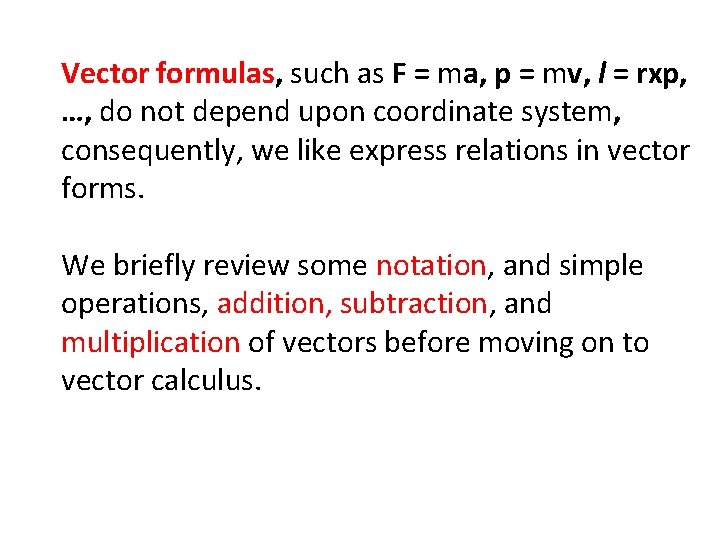Vector formulas, such as F = ma, p = mv, l = rxp, …, do not depend upon coordinate system, consequently, we like express relations in vector forms. We briefly review some notation, and simple operations, addition, subtraction, and multiplication of vectors before moving on to vector calculus.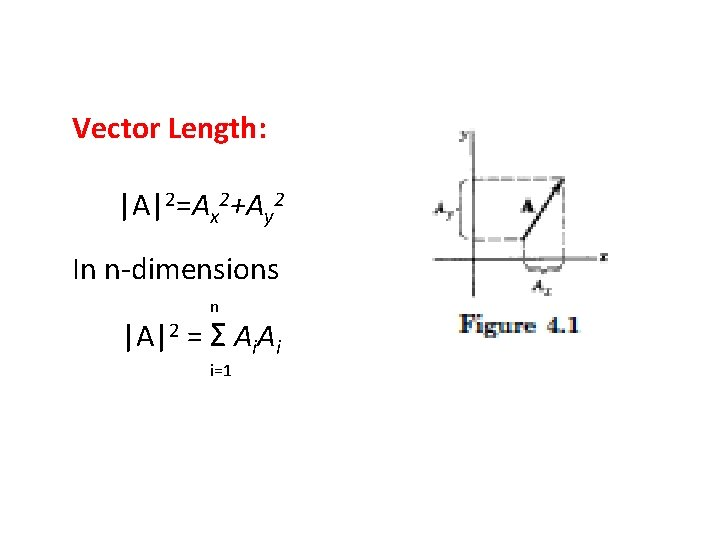Vector Length: |A|2=Ax 2+Ay 2 In n-dimensions n |A|2 = Σ Ai. Ai i=1Vector Addition: A+B=(Ax+Bx, Ay+By, Az+Bz) Commets: • A+B=B+A, commutative • A+B+C =(A+B)+C=A+(B+C),Vector Subtraction: A-B=(Ax-Bx, Ay-By, Az-Bz) Comments: • A-B=A+(-B)=(-B)+A • -A-B-C=(-A-B)-C=-A+(-B-C)Vector Multiplication by a constant: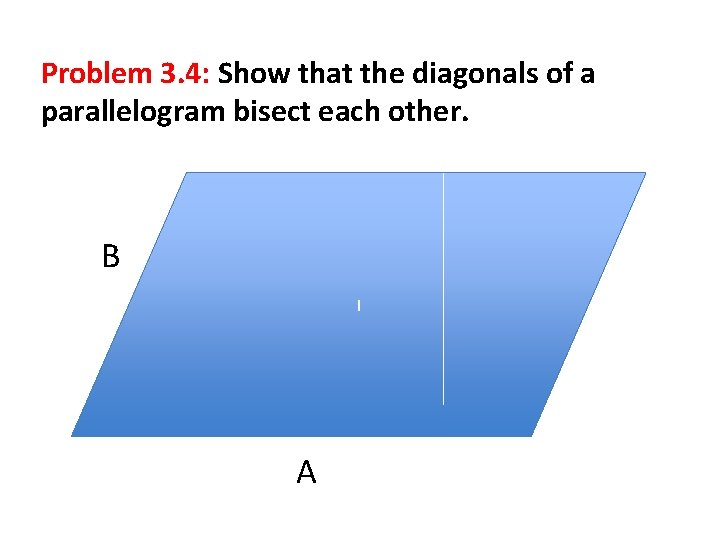Problem 3. 4: Show that the diagonals of a parallelogram bisect each other. B I A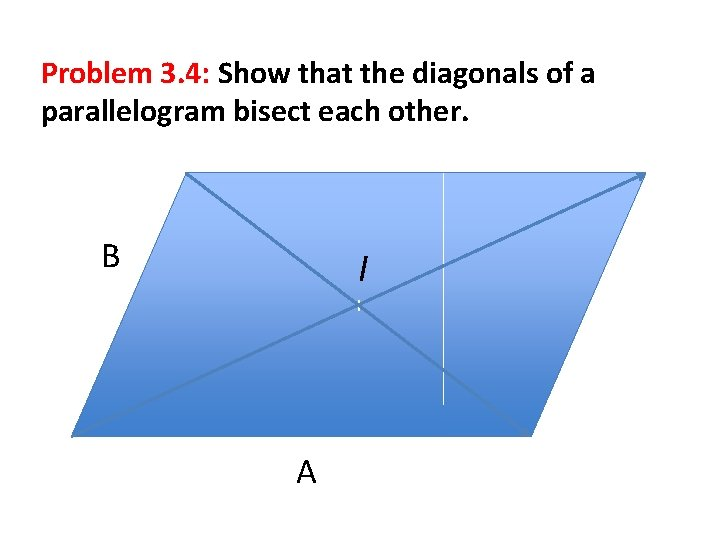Problem 3. 4: Show that the diagonals of a parallelogram bisect each other. B I I AProblem 3. 4: Show that the diagonals of a parallelogram bisect each other. A+B B I I AProblem 3. 4: Show that the diagonals of a parallelogram bisect each other. A+B B I I A-B AWrite two vectors, one approaching from the left and one from the right, IL = B+α(A-B) and IR = (A+B)-β(A+B) where α > 0 and β > 0. Note that IL = IR and solve for α and β. This leads to α = β = ½. Could also do this problem algebraically.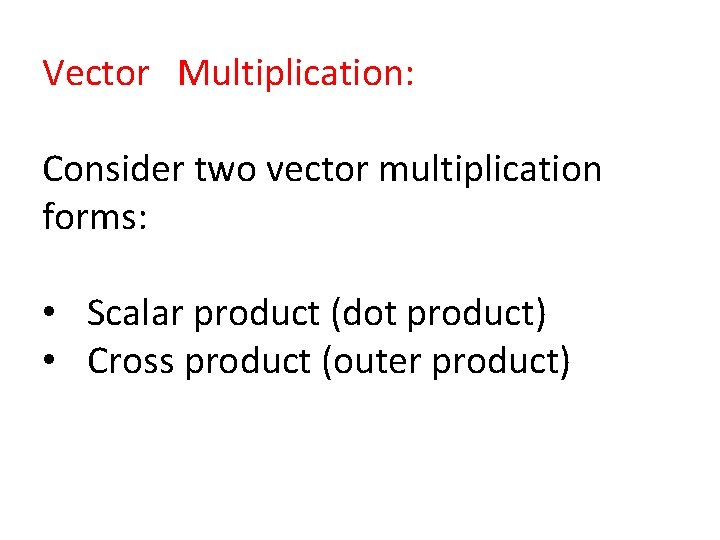Vector Multiplication: Consider two vector multiplication forms: • Scalar product (dot product) • Cross product (outer product)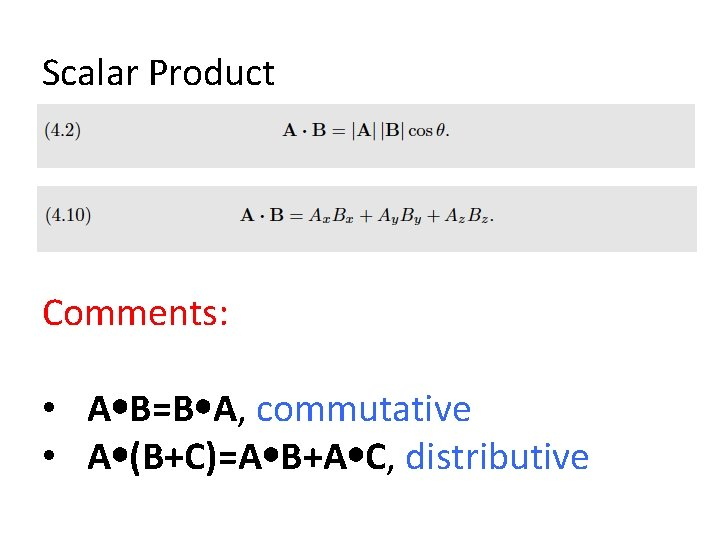Scalar Product Comments: • A B=B A, commutative • A (B+C)=A B+A C, distributiveScalar Product Comments: • A B=B A, commutative • A (B+C)=A B+A C, distributive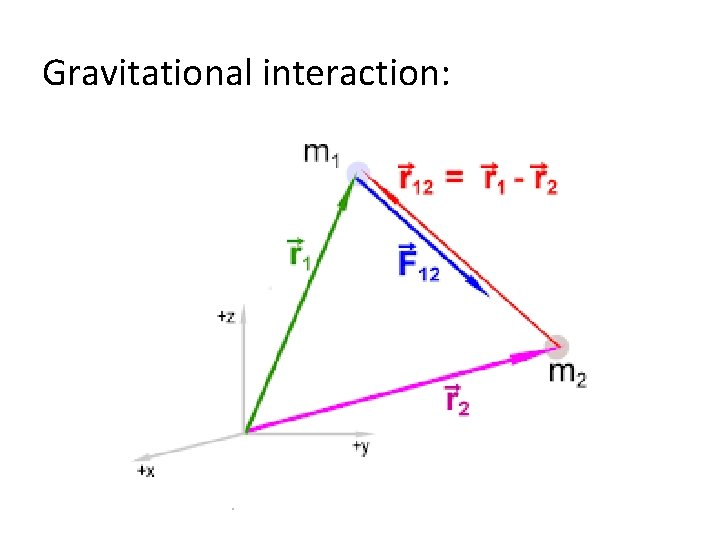Gravitational interaction: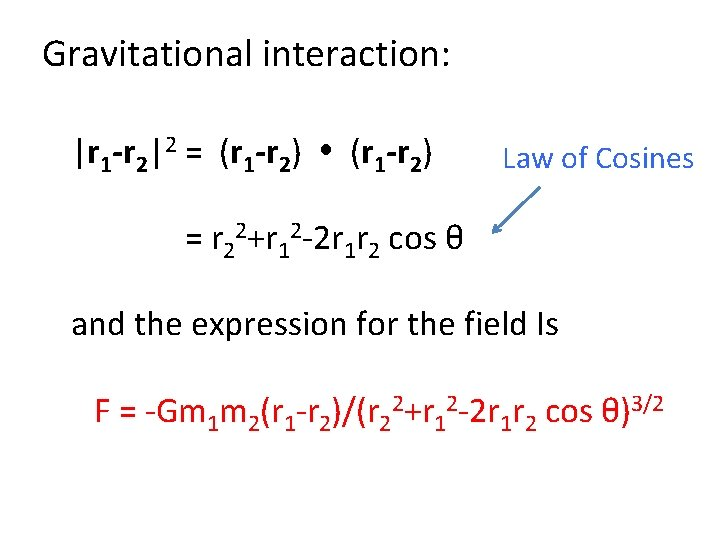Gravitational interaction: |r 1 -r 2|2 = (r 1 -r 2) Law of Cosines = r 22+r 12 -2 r 1 r 2 cos θ and the expression for the field Is F = -Gm 1 m 2(r 1 -r 2)/(r 22+r 12 -2 r 1 r 2 cos θ)3/2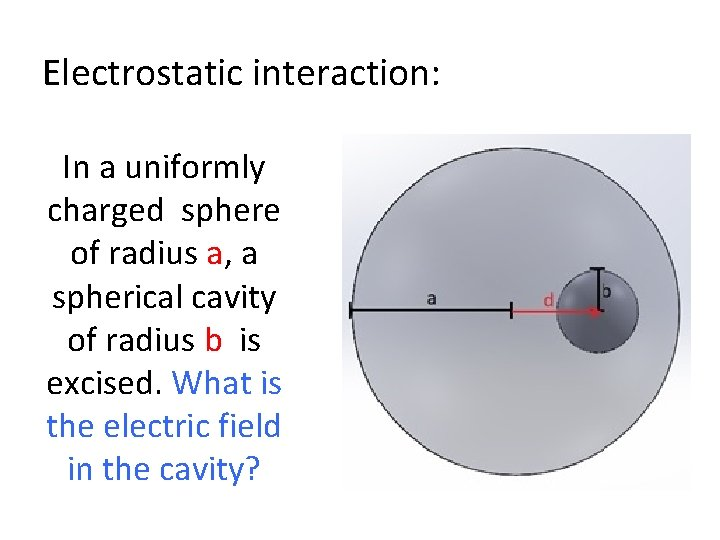Electrostatic interaction: In a uniformly charged sphere of radius a, a spherical cavity of radius b is excised. What is the electric field in the cavity?What is the electric field in the cavity? We know (can show) that the electric field in an uniformly charged sphere is radial and given by E = Q(r)r/r 3 = (4πr 3ρ/3) r/r 3 = (4πρ/3)r Field is radial and increases with r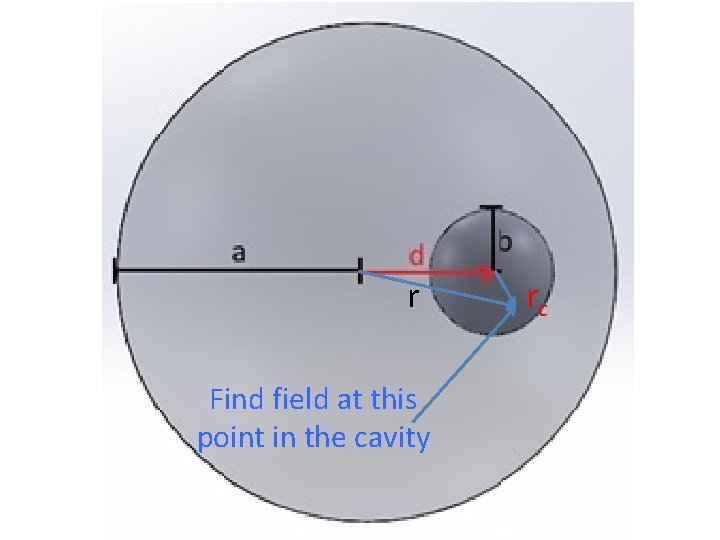r Find field at this point in the cavity rcAdd fields r rc Total = (4πρ/3)r – (4πρ/3)rc = (4πρ/3){r-(r-d)} = (4πρ/3)d Field Is uniform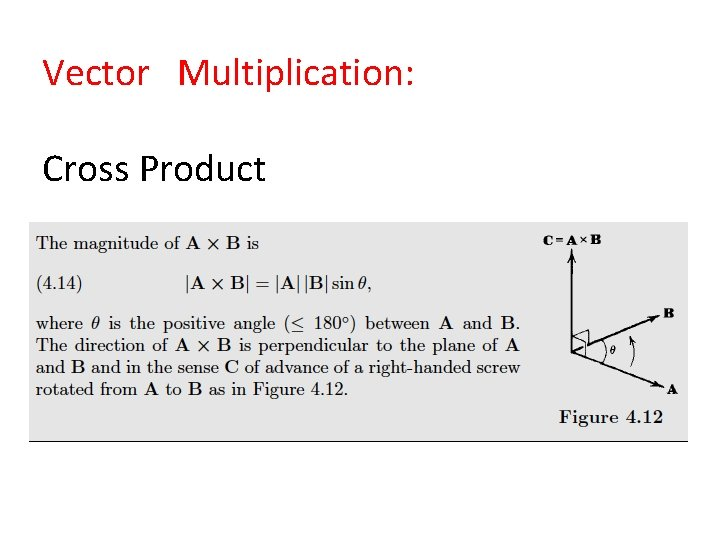Vector Multiplication: Cross Product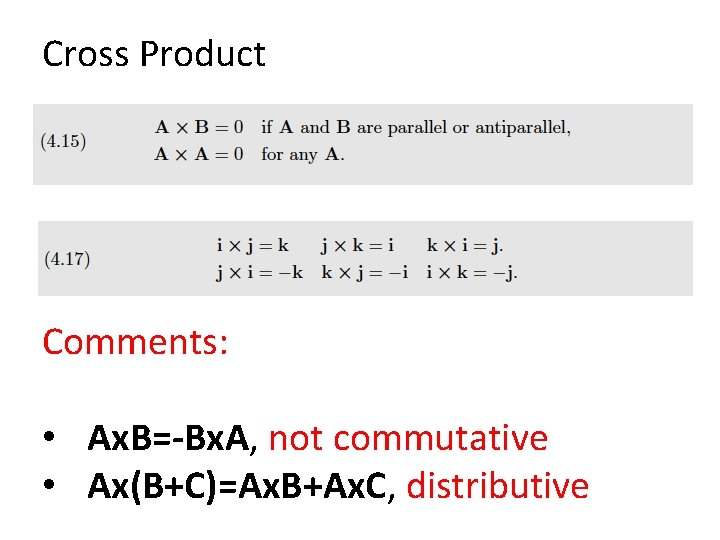Cross Product Comments: • Ax. B=-Bx. A, not commutative • Ax(B+C)=Ax. B+Ax. C, distributive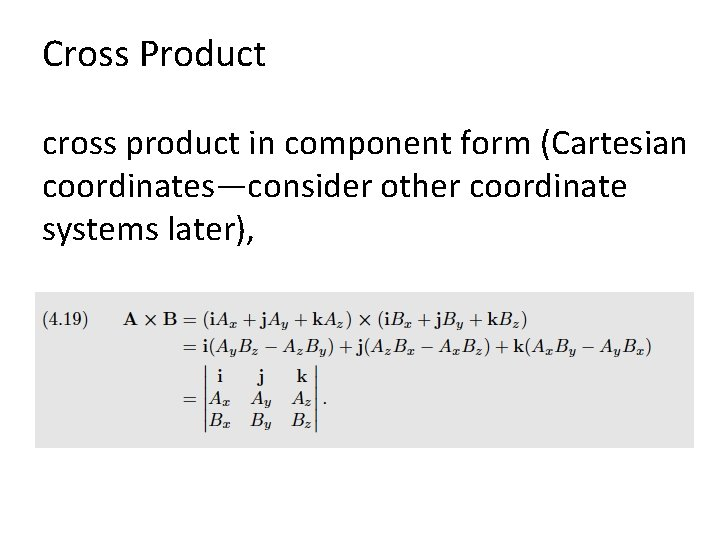Cross Product cross product in component form (Cartesian coordinates—consider other coordinate systems later),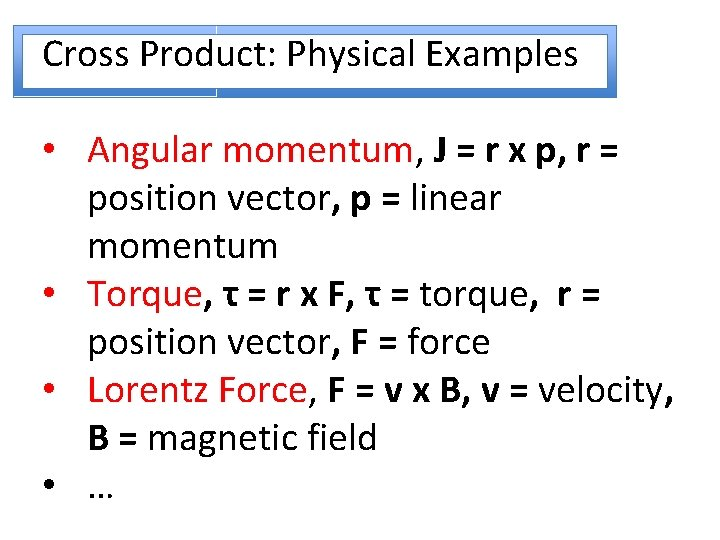Cross Product: Physical Examples • Angular momentum, J = r x p, r = position vector, p = linear momentum • Torque, τ = r x F, τ = torque, r = position vector, F = force • Lorentz Force, F = v x B, v = velocity, B = magnetic field • …Consider a rotating object: The Earth spins on its axis with rotation period P= 23 h 56. 091 s. What is the speed of an object fixed to the Earth at latitude λ = (½π- θ)? θ is the polar angle. The object moves a distance D = 2πr sin θ per rotation of the Earth. Its speed is then |v| = D/P = 2πr sin θ x (|Ω|/2π) = r|Ω|sin θ v = Ω x r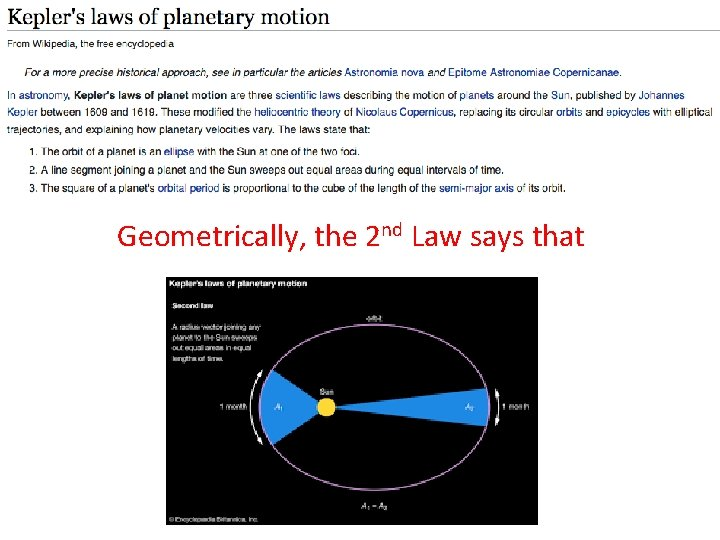Geometrically, the 2 nd Law says thatwe construct Sun r |v|dt PlanetArea, A θ Form the parallelogram and find the area A, First find the area of the rectangle, (r+|v|dt cosθ) |v|dt sinθArea, A θ Form the parallelogram and find the area A, Next, subtract the triangles to find 2 A, 2 A = (r+|v|dt cosθ) |v|dt sinθ - |v|2 dt 2 (cosθ sinθ)Area, A θ Form the parallelogram and find the area A, Next, subtract the triangles to find 2 A, 2 A = (r+|v|dt cosθ) |v|dt sinθ - |v|dt (cosθ sinθ) This yields, 2 A = r|v|dt sinθ 2 A/dt = r|v|sinθArea, A θ If the planet has mass m, then 2 m. A/dt = r|mv|sinθ = |r x mv| = |r x p| = |J| Kepler’s 2 nd says that the area, A, swept out for any time interval dt is constant so that, |J| = constantB θ A In general, the area of the triangle, sides A and B where A and B form angle θ, is given by Area = ½|A x B |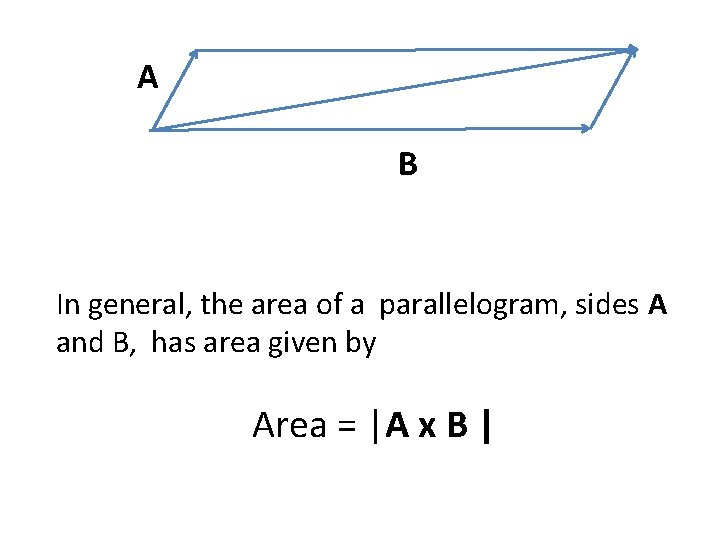A B In general, the area of a parallelogram, sides A and B, has area given by Area = |A x B |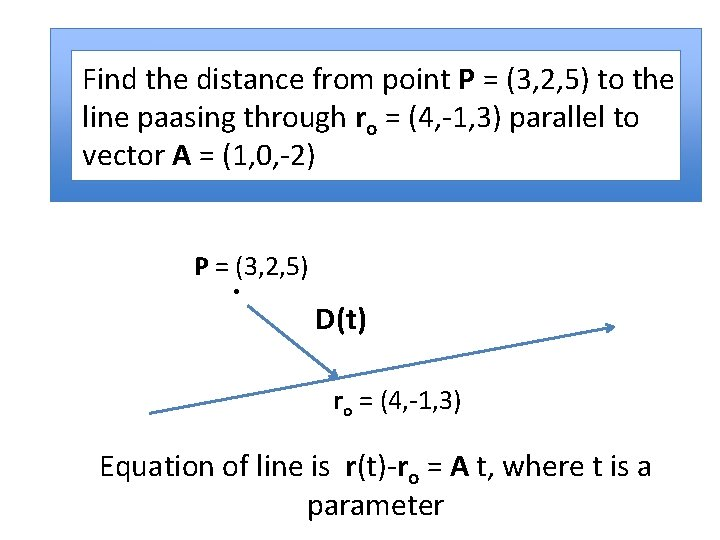Find the distance from point P = (3, 2, 5) to the line paasing through ro = (4, -1, 3) parallel to vector A = (1, 0, -2) P = (3, 2, 5) D(t) ro = (4, -1, 3) Equation of line is r(t)-ro = A t, where t is a parameterFind the distance from point P = (3, 2, 5) to the line paasing through ro = (4, -1, 3) parallel to vector A = (1, 0, -2) The vector between P and r(t) is D = P – r and we want the minimum value for this length, that is, we want to find |D| when D and A are perpendicular, that is, when D A=0Find the distance from point P = (3, 2, 5) to the line passing through ro = (4, -1, 3) parallel to vector A = (1, 0, -2) D A = ( P – r ) A = (P – ro-A t) A = 0 when (P-ro) A–A A t = 0 so t = (-5)/(5) = -1 and |Dmin| = 3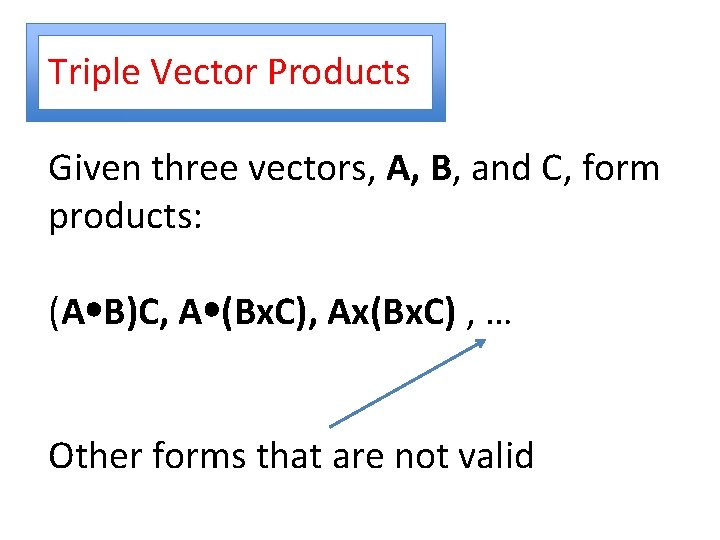Triple Vector Products Given three vectors, A, B, and C, form products: (A B)C, A (Bx. C), Ax(Bx. C) , … Other forms that are not valid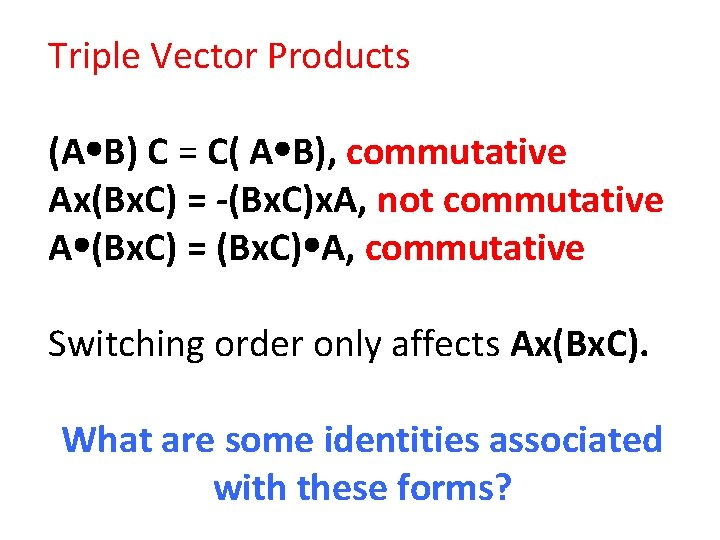Triple Vector Products (A B) C = C( A B), commutative Ax(Bx. C) = -(Bx. C)x. A, not commutative A (Bx. C) = (Bx. C) A, commutative Switching order only affects Ax(Bx. C). What are some identities associated with these forms?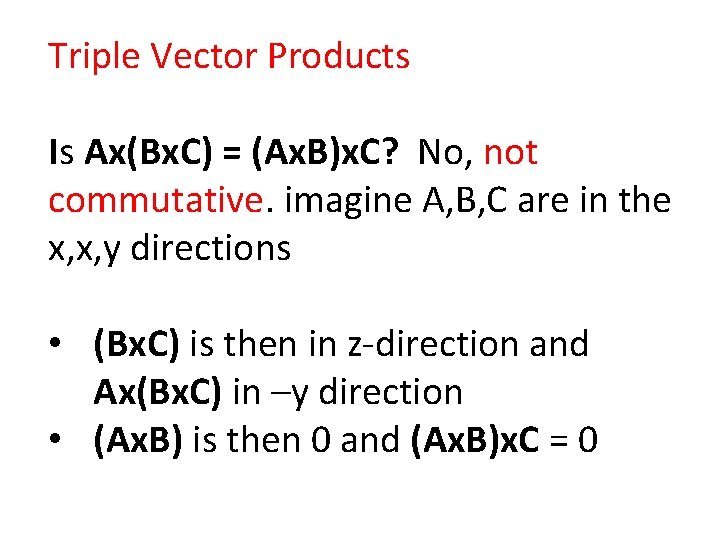Triple Vector Products Is Ax(Bx. C) = (Ax. B)x. C? No, not commutative. imagine A, B, C are in the x, x, y directions • (Bx. C) is then in z-direction and Ax(Bx. C) in –y direction • (Ax. B) is then 0 and (Ax. B)x. C = 0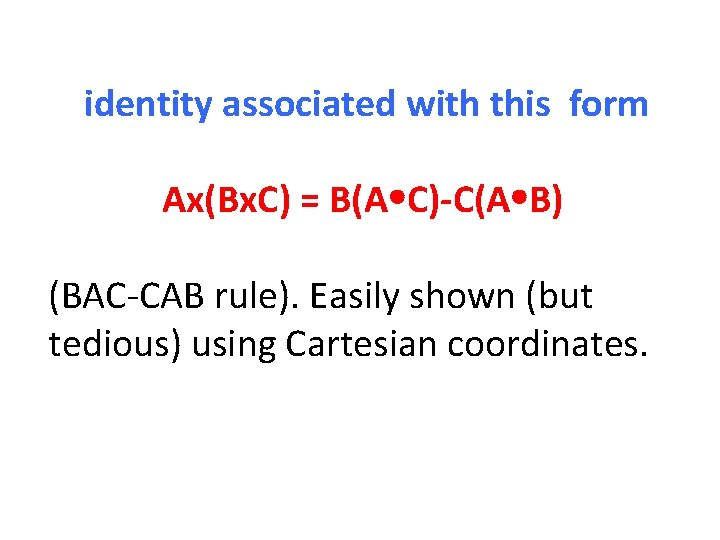identity associated with this form Ax(Bx. C) = B(A C)-C(A B) (BAC-CAB rule). Easily shown (but tedious) using Cartesian coordinates.Ax(Bx. C) = B(A C)-C(A B) (Bx. C) =(Bx, By, Bz)x(Cx, Cy, Cz) = (By. Cz-Bz. Cy, Bz. Cx-Bx. Cz, Bx. Cy-By. Cx) = (fx, fy, fz) Ax(Bx. C) = (Ayfz-Azfy, Azfx-Axfz, Axfy-Ayfx)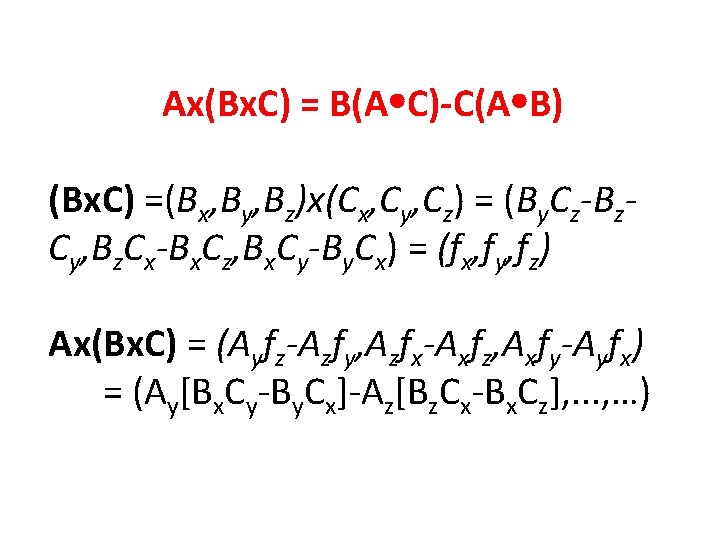Ax(Bx. C) = B(A C)-C(A B) (Bx. C) =(Bx, By, Bz)x(Cx, Cy, Cz) = (By. Cz-Bz. Cy, Bz. Cx-Bx. Cz, Bx. Cy-By. Cx) = (fx, fy, fz) Ax(Bx. C) = (Ayfz-Azfy, Azfx-Axfz, Axfy-Ayfx) = (Ay[Bx. Cy-By. Cx]-Az[Bz. Cx-Bx. Cz], . . . , …)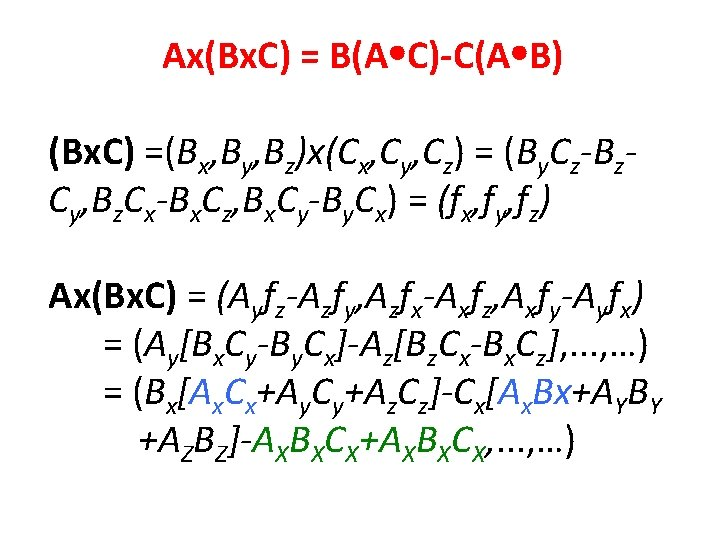Ax(Bx. C) = B(A C)-C(A B) (Bx. C) =(Bx, By, Bz)x(Cx, Cy, Cz) = (By. Cz-Bz. Cy, Bz. Cx-Bx. Cz, Bx. Cy-By. Cx) = (fx, fy, fz) Ax(Bx. C) = (Ayfz-Azfy, Azfx-Axfz, Axfy-Ayfx) = (Ay[Bx. Cy-By. Cx]-Az[Bz. Cx-Bx. Cz], . . . , …) = (Bx[Ax. Cx+Ay. Cy+Az. Cz]-Cx[Ax. Bx+AYBY +AZBZ]-AXBXCX+AXBXCX, . . . , …)Ax(Bx. C) = B(A C)-C(A B) Ax(Bx. C) = (Ayfz-Azfy, Azfx-Axfz, Axfy-Ayfx) = (Ay[Bx. Cy-By. Cx]-Az[Bz. Cx-Bx. Cz], . . . , …) = (Bx[Ax. Cx+Ay. Cy+Az. Cz]-Cx[Ax. Bx+AYBY +AZBZ]-AXBXCX+AXBXCX, . . . , …) Generalize to B(A C) – C(A B)identity associated with this form (Ax. B)x. C = -Cx(Ax. B) = -A(C B)+B(C A)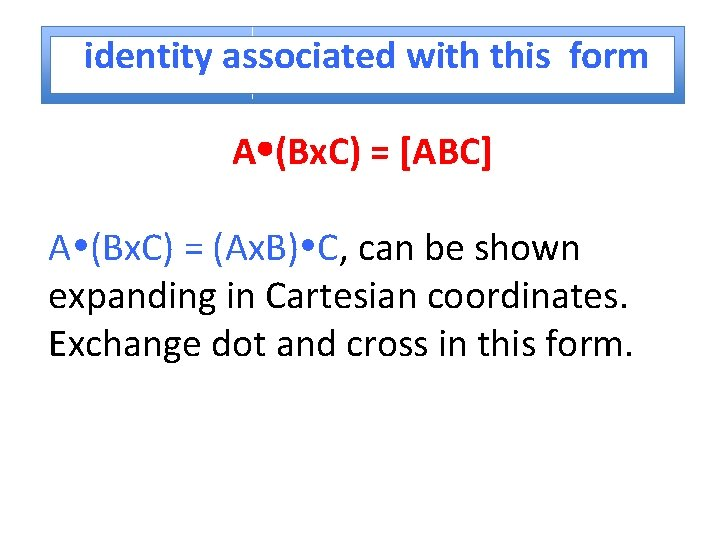identity associated with this form A (Bx. C) = [ABC] A (Bx. C) = (Ax. B) C, can be shown expanding in Cartesian coordinates. Exchange dot and cross in this form.identity associated with this form A (Bx. C) = (Ax, Ay, Az)(fx, fy, fz) = Ax(By. Cz-Bz. Cy)+Ay(Bz. Cx-Bx. Cz) +Az(Bx. Cy-By. Cx) A (Bx. C) = Cx(Ay. Bz-Az. By)+Cy(Az. Bx-Ax. Bz) +Cz(Ax. By-Ay. Bx) = (Ax. B) C = C (Ax. B)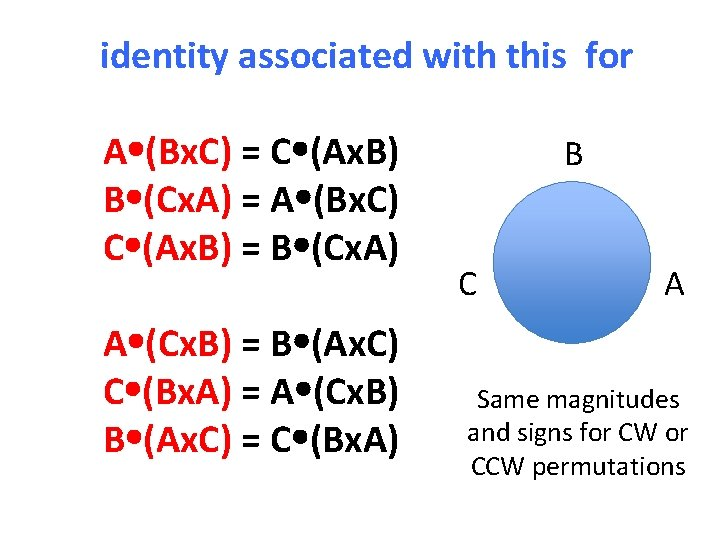identity associated with this for A (Bx. C) = C (Ax. B) B (Cx. A) = A (Bx. C) C (Ax. B) = B (Cx. A) A (Cx. B) = B (Ax. C) C (Bx. A) = A (Cx. B) B (Ax. C) = C (Bx. A) B C A Same magnitudes and signs for CW or CCW permutationsConsider vectors, A, B, C; A, B, C are not all parallel to the same plane, [ABC] ≠ 0 Show that any vector can be written as a linear combination of A, B, & C.Let some vector V, be expressed as V = a. A + b. B +c. C Cross V into B, Vx. B = a. Ax. B + c. Cx. B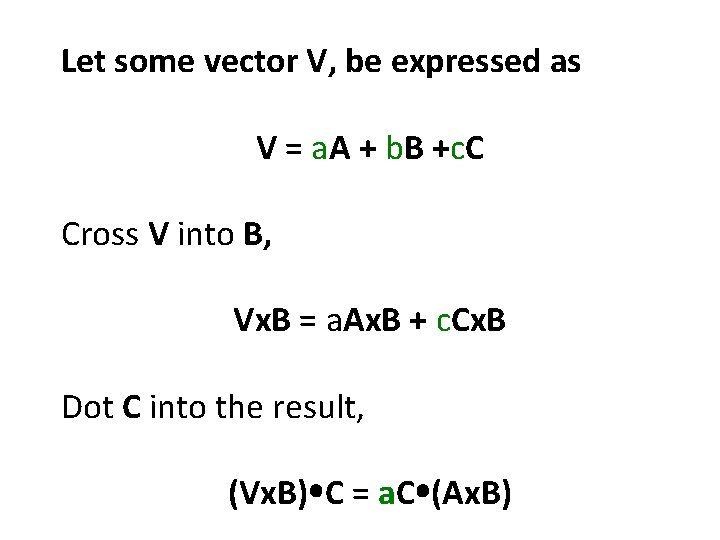Let some vector V, be expressed as V = a. A + b. B +c. C Cross V into B, Vx. B = a. Ax. B + c. Cx. B Dot C into the result, (Vx. B) C = a. C (Ax. B)Solve for a a = (Vx. B) C / C (Ax. B)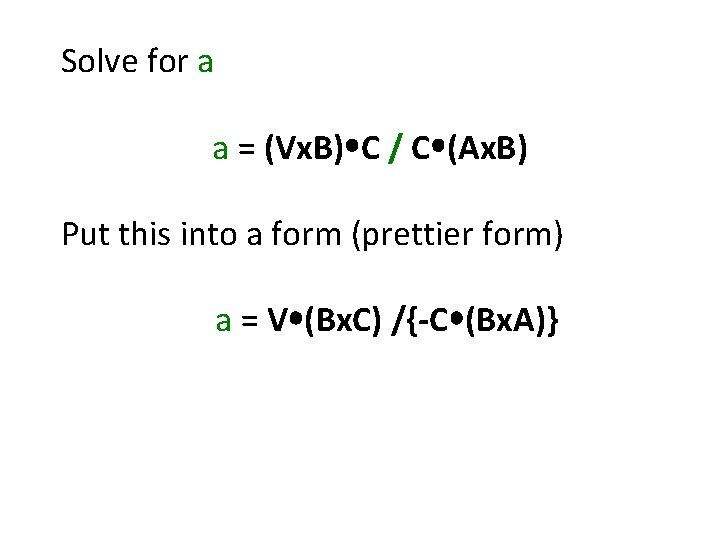Solve for a a = (Vx. B) C / C (Ax. B) Put this into a form (prettier form) a = V (Bx. C) /{-C (Bx. A)}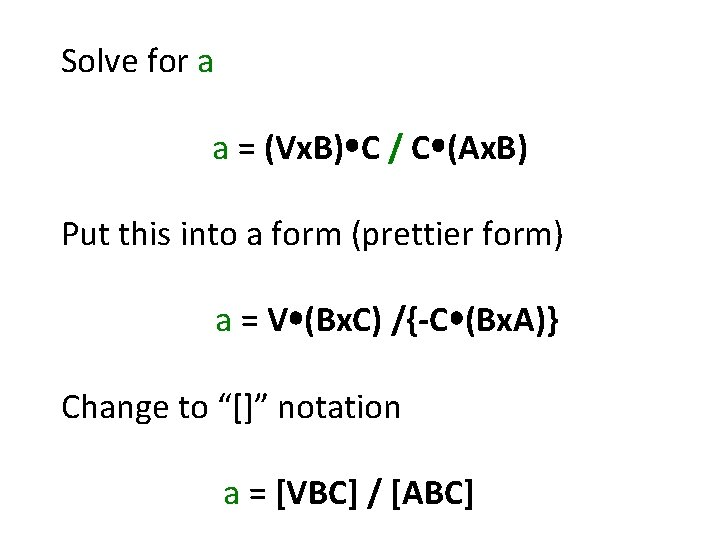Solve for a a = (Vx. B) C / C (Ax. B) Put this into a form (prettier form) a = V (Bx. C) /{-C (Bx. A)} Change to “[]” notation a = [VBC] / [ABC]Similarly for b and c leads to a = [VBC] / [ABC] b = [AVC] / [ABC] c = [ABV] / [ABC]